# AP Physics C: Mechanics Practice Test 5

### Test Information10 questions13 minutes

1.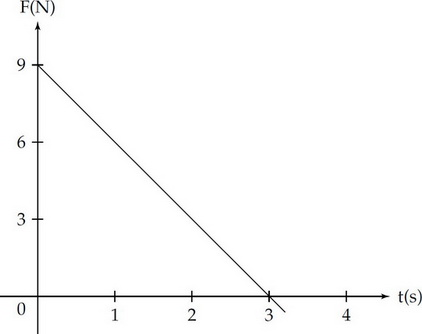A block of mass 2 kg, initially at rest, is pulled along a frictionless, horizontal surface with a force shown as a function of time by the graph above.

The speed of the block at t = 3 s is

2.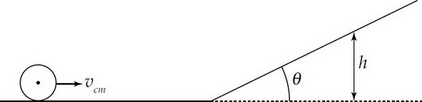The center of mass of a cylinder of mass m, radius r, and rotational inertia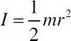has a velocity of vcm as it rolls without slipping along a horizontal surface. It then encounters a ramp of angle θ, and continues to roll up the ramp without slipping.

What is the maximum height the cylinder reaches?

3.The center of mass of a cylinder of mass m, radius r, and rotational inertiahas a velocity of vcm as it rolls without slipping along a horizontal surface. It then encounters a ramp of angle θ, and continues to roll up the ramp without slipping.

Now the cylinder is replaced with a hoop that has the same mass and radius. The hoop's rotational inertia is mr2. The center of mass of the hoop has the same velocity as the cylinder when it is rolling along the horizontal surface and the hoop also rolls up the ramp without slipping. How would the maximum height of the hoop compare to the maximum height of the cylinder?

4.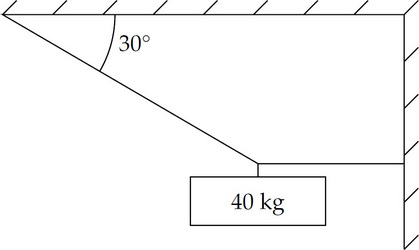An object of mass 40 kg is suspended by means of two cords, as shown above. The tension in the angled cord is

5. A box is on an incline of angle q above the horizontal. The box may be subject to the following forces: frictional (f), gravitational (Fg), tension from a string connected to it (FT) and normal (N). In the following free-body diagrams for the box, the lengths of the vectors are proportional to the magnitudes of the forces.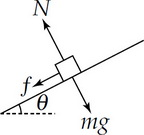Figure A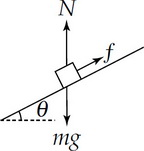Figure B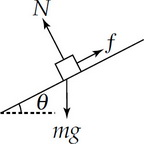Figure C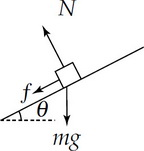Figure D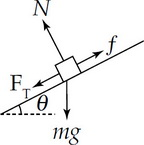Figure E

Which of the following best represents the free-body diagram for the box if it is decelerating as it goes up the incline?

6. A box is on an incline of angle q above the horizontal. The box may be subject to the following forces: frictional (f), gravitational (Fg), tension from a string connected to it (FT) and normal (N). In the following free-body diagrams for the box, the lengths of the vectors are proportional to the magnitudes of the forces.Figure AFigure BFigure CFigure DFigure E

Which of the following best represents the free-body diagram for the box if it is moving at a constant velocity down the ramp?

7. A box is on an incline of angle q above the horizontal. The box may be subject to the following forces: frictional (f), gravitational (Fg), tension from a string connected to it (FT) and normal (N). In the following free-body diagrams for the box, the lengths of the vectors are proportional to the magnitudes of the forces.Figure AFigure BFigure CFigure DFigure E

Which of the following best represents the free-body diagram for the box if its speed is increasing as it moves down the incline?

8.The force on an object as a function of time t is given by the expression F = Ct3, where C is a constant. Determine the change in momentum for the time interval 0 to t1.

9.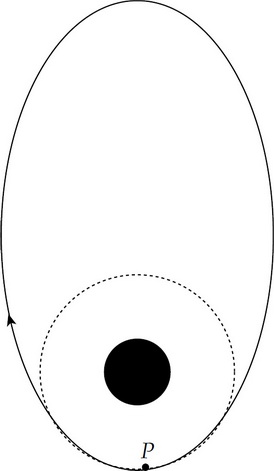A spaceship orbits Earth in a clockwise, elliptical orbit as shown above. The spaceship needs to change to a circular orbit. When the spaceship passes point P, a short burst of the ship's engine will change its orbit. What direction should the engine burst be directed?

10. A motorcycle of mass 200 kg completes a vertical, circular loop of radius 5 m, with a constant speed of 10 m/s. How much work is done on the motorcycle by the normal force of the track?